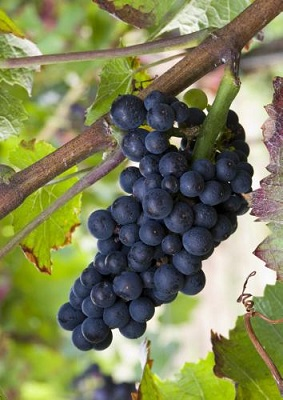• 按字母检索葡萄品种
• A
• B
• C
• D
• E
• F
• G
• H
• I
• J
• K
• L
• M
• N
• O
• P
• Q
• R
• S
• T
• U
• V
• W
• X
• Y
• Z

内格瑞特(Negrette)Cape de More, Degoutant, Morelet, Morillon, Mourrelet, Negralet, Negret, Negret de Gaillac, Negret du Tarn, Negrette de Fronton, Noirien, Pinot St George, Vesparo Noir, Ragoutant